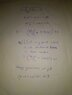# Rings on a rotating rod

• Divya
This equation is very easy to understand once you understand that the parameter x is the distance between the rings and the center, and that the factor 0.9 works because the rings are moving away from the center at a speed of 0.9 meters per second.Thank you very much.f

#### Divya

Homework Statement
A rigid horizontal smooth rod $AB$ of mass $0.75 kg$ and length $40 cm$ can rotate freely about a fixed vertical axis through its mid point $O$. Two rings each of mass $1 Kg$ are initially at rest at a distance of $10 cm$ from $O$ on the either side of the rod.The rod is set in rotation with an angular velocity of $30$ radians per second. Find the velocity of each ring along the length of the rod in m/s when they reach the ends of the rod.
Relevant Equations
(I am new to this forum, I don't know what to write in 'Relevant Equations' field)
Method 1: Simply conserving angular momentum about the the fixed vertical axis and conserving energy gives ##v=3##, which is correct according to my book.

Method 2: Conserving angular momentum when the two rings reach distance ##x## from the centre gives
##(0.01+2x^2) \omega =0.9##
Also in the rod's frame ##a=v dv/dx =\omega ^2 x## (where a and v radial acceleration and velocities).
So, ##v^2/2=\int_{0.1}^{0.2} \frac {0.9xdx} {(0.01+2x^2)^2}=5##.
So, ##v=\sqrt{10}##.
What is wrong in second method?

Edit: Everything is in SI unit

Last edited:
The angular velocity of the rod decreases as the rings move outwards. The formula you used in the second method doesn't work with a reference frame that changes its angular velocity.

There are a lot of units missing.

Why? The fictitious forces are centrifugal, coriolis and azimuthal. Here only centrifugal force is in radial direction. So, the equation should be correct.

The angular velocity of the rod decreases as the rings move outwards. The formula you used in the second method doesn't work with a reference frame that changes its angular velocity.

There are a lot of units missing.
Sorry, everything is in SI unit.

These are the forces in a reference frame where the angular velocity stays the same. I guess you could try to do that with the initial angular velocity but it just makes things needlessly complicated.
Sorry, everything is in SI unit.
And it should be added to the quantities that have units. It's a bad habit to not do that, especially combined with equations where quantities are not introduced first. 0.01 is what? Sure, it's possible to guess it from context, but it's just unnecessary. Don't let others guess.

These are the forces in a reference frame where the angular velocity stays the same. I guess you could try to do that with the initial angular velocity but it just makes things needlessly complicated.And it should be added to the quantities that have units. It's a bad habit to not do that, especially combined with equations where quantities are not introduced first. 0.01 is what? Sure, it's possible to guess it from context, but it's just unnecessary. Don't let others guess.
As far I know, in case of non-uniformly rotating frame, everything is same except that we also need to add azimuthal force. And here azimuthal force is not in radial direction.

##(0.01+2x^2) \omega =0.9##
Plugging in numbers straight away and with no text explanation makes it hard to follow the reasoning. Please explain the derivation of this equation. I was expecting ##x^2\omega=x_0^2\omega_0##.

@haruspex, I have added the explanation of this equation in this attachment.

#### Attachments

•16021384310777060270523105675145.jpg
30 KB · Views: 85
@haruspex, I have added the explanation of this equation in this attachment.
Ok, thanks.
Your error is that you forgot to square the 0.9.

Ok, thanks.
Your error is that you forgot to square the 0.9.
I don't think so.

##(0.01+2x^2) \omega =0.9##
So, ##v^2/2=\int_{0.1}^{0.2} \frac {0.9xdx} {(0.01+2x^2)^2}=5##.
## \omega =\frac {0.9}{0.01+2x^2}##
## \omega ^2=\frac {0.9^2}{(0.01+2x^2)^2}##

Thank you very much.